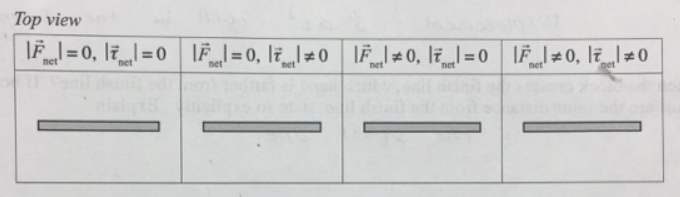# Problem: A uniform rigid rod rests on a level, frictionless surface. The diagram below indicates four different combinations of (1) net force on the rod, Fnet, and (2) net torque on the rod about its center of mass, tnet. In each box, draw vectors that represent one or two forces that achieve the given combination of net force and net torque. If any combination is not possible, state so explicitly.For example: In the second case, indicate one or two forces that could be exerted on the rod so that at the instant shown the net force on it is zero, but the net torque on it is not zero.

###### FREE Expert Solution

Torque is a rotational motion.

If τnet = 0 and |Fnet| = 0, it means that the external forces cancel.

Therefore, one external force will be going up while the other is going down.

90% (211 ratings)###### Problem Details

A uniform rigid rod rests on a level, frictionless surface. The diagram below indicates four different combinations of (1) net force on the rod, Fnet, and (2) net torque on the rod about its center of mass, tnet. In each box, draw vectors that represent one or two forces that achieve the given combination of net force and net torque. If any combination is not possible, state so explicitly.For example: In the second case, indicate one or two forces that could be exerted on the rod so that at the instant shown the net force on it is zero, but the net torque on it is not zero.